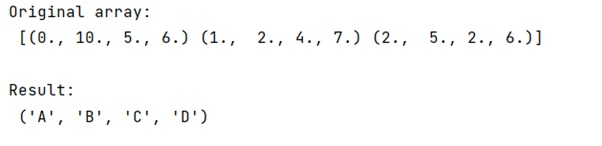# Get the column names of a NumPy ndarray

Learn how to Get the column names of a python NumPy ndarray in Python?
Submitted by Pranit Sharma, on February 09, 2023

NumPy is an abbreviated form of Numerical Python. It is used for different types of scientific operations in python. Numpy is a vast library in python which is used for almost every kind of scientific or mathematical operation. It is itself an array which is a collection of various methods and functions for processing the arrays.

## NumPy Array - Getting the column names

Suppose that we are given a .txt file that we want to load and generate a numpy array from it. This txt file contains a dataset in the form of rows and columns and each column has its name.

When we print our created array, it will not show the column names instead, we need to index the array with the column name.
Assuming that the column names may vary from time to time, it is difficult to index the array without knowing the name of the column hence, we need to find a way to get the names of the columns of ndarray.

For this purpose, we will use dtype.names() with the created array and this will return an array of all the names of the columns.

Let us understand with the help of an example,

## Python code to get the column names of a NumPy ndarray

```# Import numpy
import numpy as np

# Creating a numpy array
arr =  np.genfromtxt("data.txt",names=True)

# Display original array
print("Original array:\n",arr,"\n")

# Getting column names
res = arr.dtype.names

# Display Result
print("Result:\n",res,"\n")
```

Output: